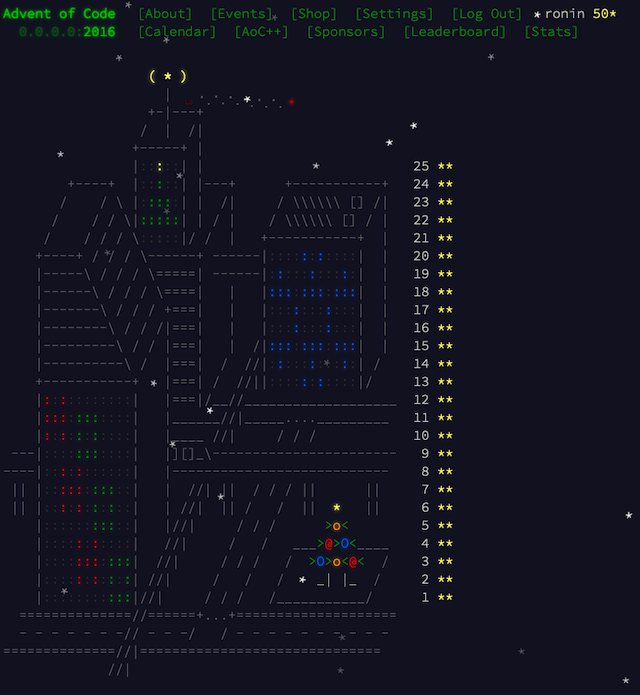# Advent of Code 2016, Day 25: Clock Signal

## Part A

On Day 25 we need to work with assembunny code again. We have our input program:

``````cpy a d
cpy 15 c
cpy 170 b
inc d
dec b
jnz b -2
dec c
jnz c -5
cpy d a
jnz 0 0
cpy a b
cpy 0 a
cpy 2 c
jnz b 2
jnz 1 6
dec b
dec c
jnz c -4
inc a
jnz 1 -7
cpy 2 b
jnz c 2
jnz 1 4
dec b
dec c
jnz 1 -4
jnz 0 0
out b
jnz a -19
jnz 1 -21
``````

And it is supposed to transmit clock signal 0, 1, 0, 1, 0, 1 and so on forever. Emitting signal is done by `out` instruction. What we need to figure out is what is the lowest positive integer that can be used to initialize register A so such infinite signal is registered.

For that you need to reverse engineer the code. In my case there was multiplication again in the beginning. My program is multiplying 15 by 170 and then adds the result to A register. Multiplication is implement as addition, so it is better to change input program to use `mul` instruction (implemented on some other day):

``````add a 10
jnz 0 0
cpy a b
cpy 0 a
cpy 2 c
jnz b 2
jnz 1 6
dec b
dec c
jnz c -4
inc a
jnz 1 -7
cpy 2 b
jnz c 2
jnz 1 4
dec b
dec c
jnz 1 -4
jnz 0 0
out b
jnz a -19
jnz 1 -21
``````

We can reverse engineer later on. What the rest of the code is doing is diving the number in A register by 2 and then calculating the modulo. And this is send as our clock signal. So to make it alternate between 0 and 1, we need to feed it the number that will look like this `101010101010101`. In my case it was 180. 180 + 15 * 170 = 2730 = 101010101010.

Here is also the naive solution to check consecutive values for A register:

``````require "io/console"

class InvalidSignal < StandardError; end

def run(data, registers)
pc = 0

previous = nil
ticks = 0

while pc < data.size
line = data[pc]
jump = false

if line =~ /cpy (-?\d+) ([a-d])/
value = Regexp.last_match.to_i
register = Regexp.last_match

registers[register] = value
elsif line =~ /cpy ([a-d]) ([a-d])/
source_register = Regexp.last_match
target_register = Regexp.last_match

registers[target_register] = registers[source_register]
elsif line =~ /inc ([a-d])/
register = Regexp.last_match
registers[register] += 1
elsif line =~ /dec ([a-d])/
register = Regexp.last_match
registers[register] -= 1
elsif line =~ /jnz (-?\d+) (-?\d+)/
value = Regexp.last_match.to_i
offset = Regexp.last_match.to_i

if value != 0
pc += offset
jump = true
end
elsif line =~ /jnz ([a-d]) (-?\d+)/
register = Regexp.last_match
offset = Regexp.last_match.to_i

if registers[register] != 0
pc += offset
jump = true
end
elsif line =~ /jnz (-?\d+) ([a-d])/
register = Regexp.last_match
offset = registers[register]
value = Regexp.last_match.to_i

if value != 0
pc += offset
jump = true
end
elsif line =~ /tgl ([a-d])/
register = Regexp.last_match
offset = registers[register]

if data[pc + offset]
case data[pc + offset][0..2]
when "inc"
data[pc + offset][0..2] = "dec"
when "dec"
data[pc + offset][0..2] = "inc"
when "cpy"
data[pc + offset][0..2] = "jnz"
when "jnz"
data[pc + offset][0..2] = "cpy"
when "tgl"
data[pc + offset][0..2] = "inc"
end
end
elsif line =~ /out ([a-d])/
register = Regexp.last_match

if previous.nil?
if registers[register] == 0
previous = registers[register]
ticks += 1
else
raise InvalidSignal, ticks
end
else
if previous && (1 - registers[register]) != previous
raise InvalidSignal, ticks
else
previous = registers[register]
ticks += 1

return if ticks > 100
end
end
# additional instructions to execute multiplication instantly, only for 23mul.txt updated instruction set
elsif line =~ /mul ([a-d]) ([a-d])/
register_1 = Regexp.last_match
register_2 = Regexp.last_match

registers[register_1] *= registers[register_2]
elsif line =~ /nop/
elsif line =~ /add ([a-d]) (-?\d+)/
register = Regexp.last_match
value = Regexp.last_match.to_i

registers[register] += value
end

pc += 1 unless jump
end
end

a = 0
while true
begin
run(data, { "a" => a, "b" => 0, "c" => 0, "d" => 0 })
puts a
break
rescue InvalidSignal => error
a += 1
puts "a = #{a}, #{error.message}"
end
end
``````

I used program with `mul` instruction to make it run fast. When executing `out` instruction I was checking if the signal is correct for 100 ticks.

## Part B